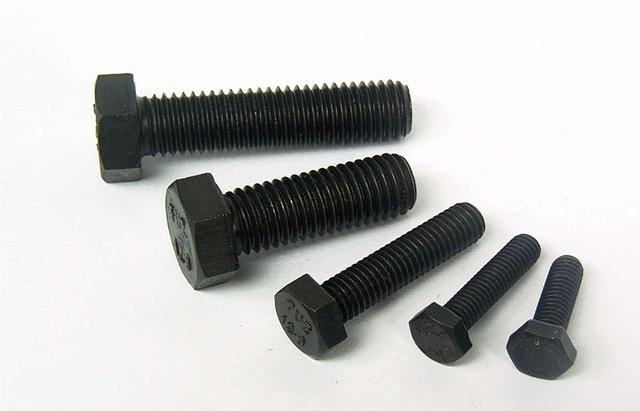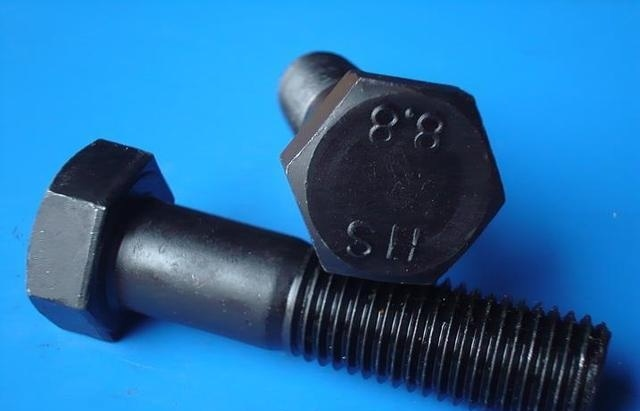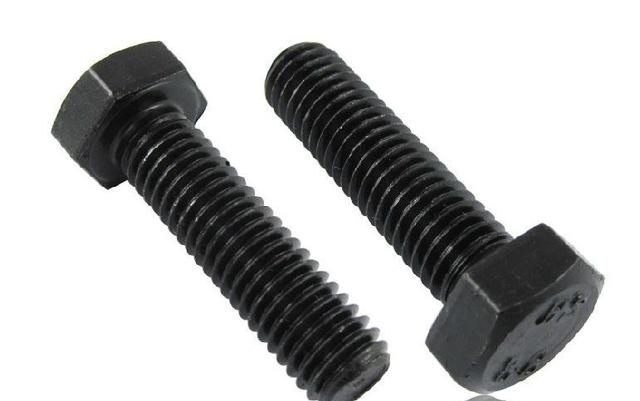# What is the meaning of the bolt's strength rating? Share it to you!

We often encounter some fasteners in our work and life. For example, the commonly used 8.8-class hex bolts, what does it mean?

Today, I would like to share with you the meaning of the strength grade of carbon steel fasteners.

The strength grade of bolts is divided into more than 10 grades of 3.6, 4.6, 4.8, 5.6, 6.8, 8.8, 9.8, 10.9, 12.9. Our daily use level is 4.8, 8.8, 10.9, 12.9.The level of the bolt represents a lot of meaning, and its grade number is composed of two parts, which respectively represent the nominal tensile strength value and the yield ratio of the bolt material.

For example, class AB bolts, the front number A represents the nominal tensile strength value of the bolt, expressed as a value of 1/100; the latter ".B" (point B) represents the ratio of the bolt yield strength, the previous value is multiplied by the latter The numerical value represents the nominal yield strength of the bolt.

AX100=Nominal tensile strength of this bolt

AX100X (B/10) = nominal yield strength of this boltFor example: 6.8 grade hex bolts have the following meanings:

1. The nominal tensile strength of the bolt is 6X100=600 Mpa=600 N/mm, ie the minimum pulling force per square millimeter is 600 N

2. The latter .8 (point 8) represents a yield strength ratio of 0.8.

3. The nominal yield strength of the bolt is =600x0.8=480Mpa= 480 N/mm, ie the yield force per square millimeter is 480 N

In general, the greater the level of the bolt, the greater the tensile strength and yield strength, and the higher the strength of the bolt.Of course, the strength grade of the bolt not only represents the above meaning, but also represents other meanings of the hardness, material, heat treatment, mechanical properties of the bolt.

Hydrophilic Fumed Silica

Hydrophilic Fumed Silica,Fumed Silica Powder,Silica Fume,Amorphous Fumed Silica

Guangzhou Quanxu Technology Co Ltd , http://www.skychema.com

Posted on